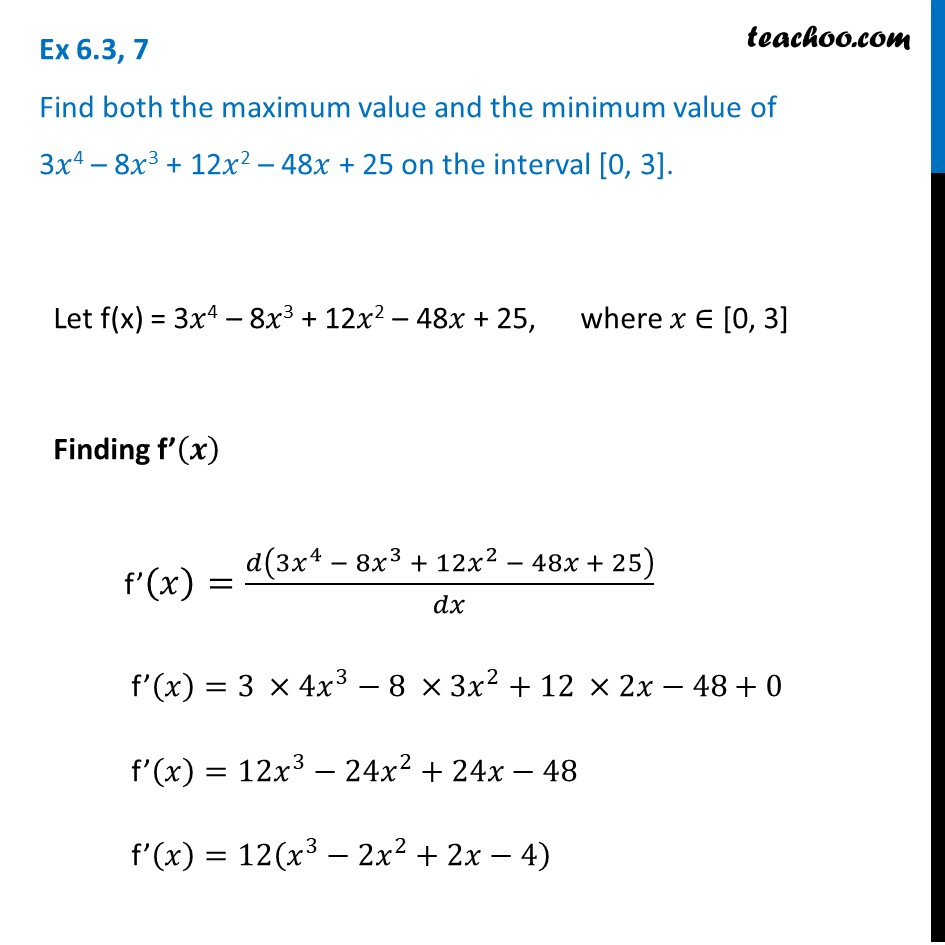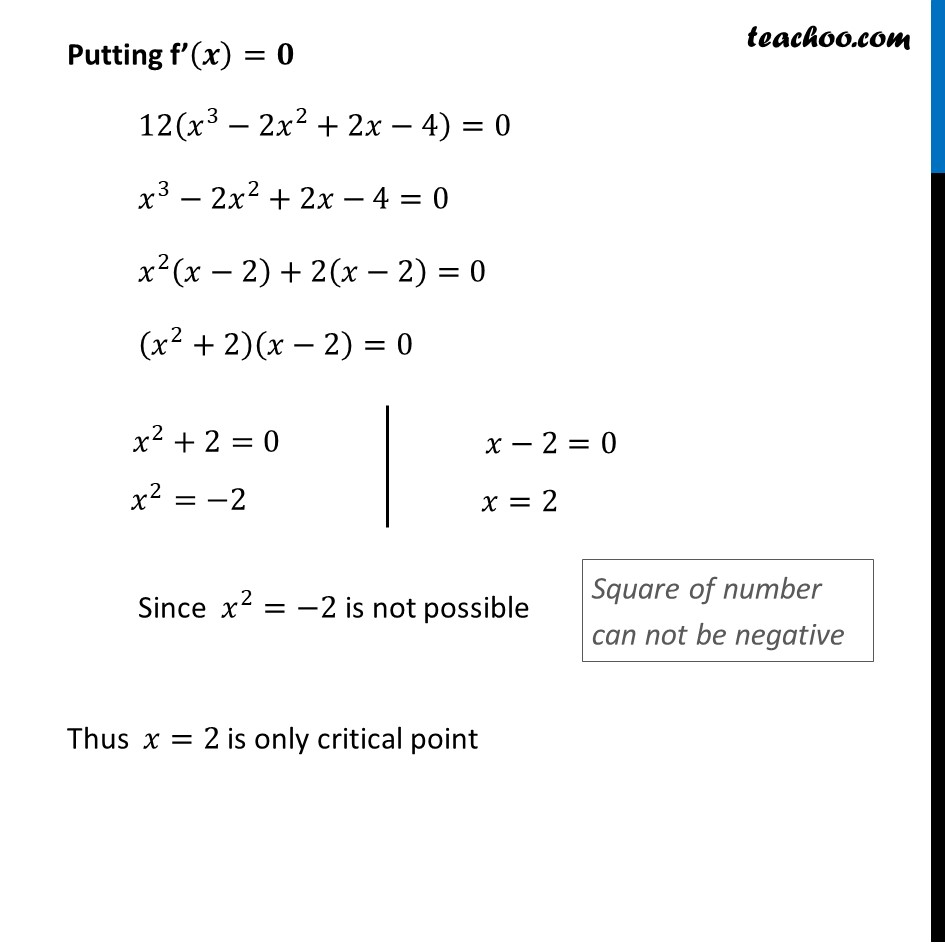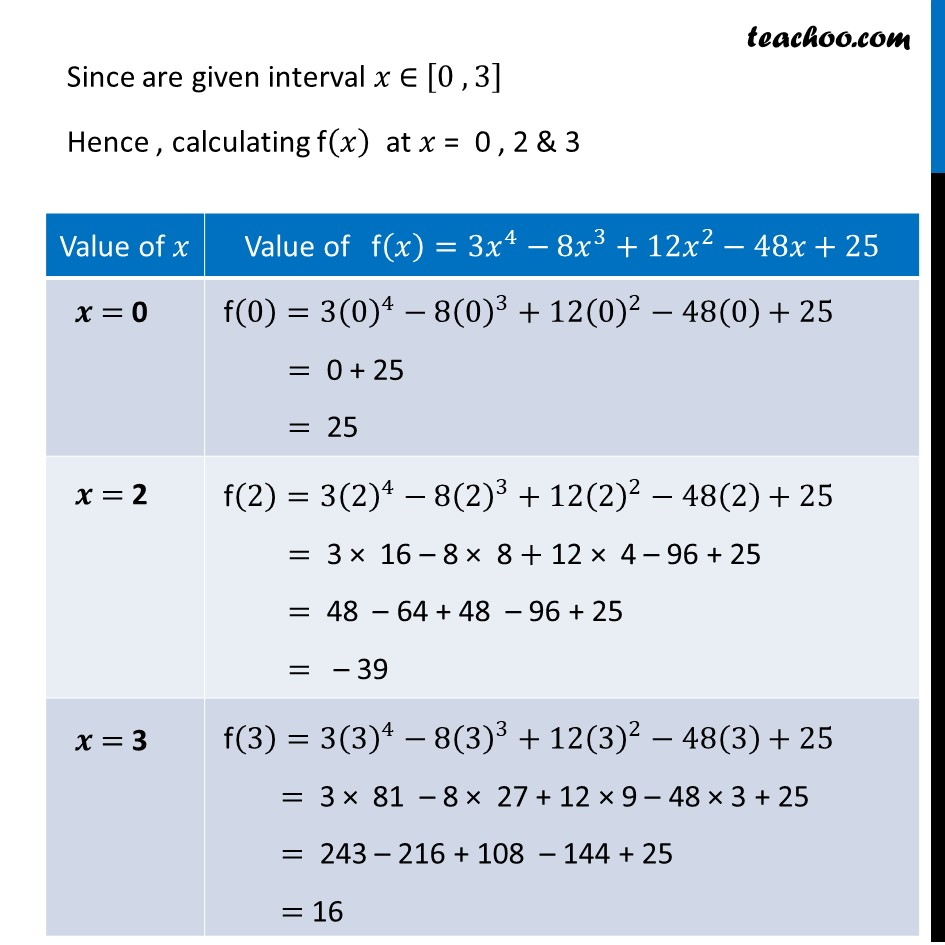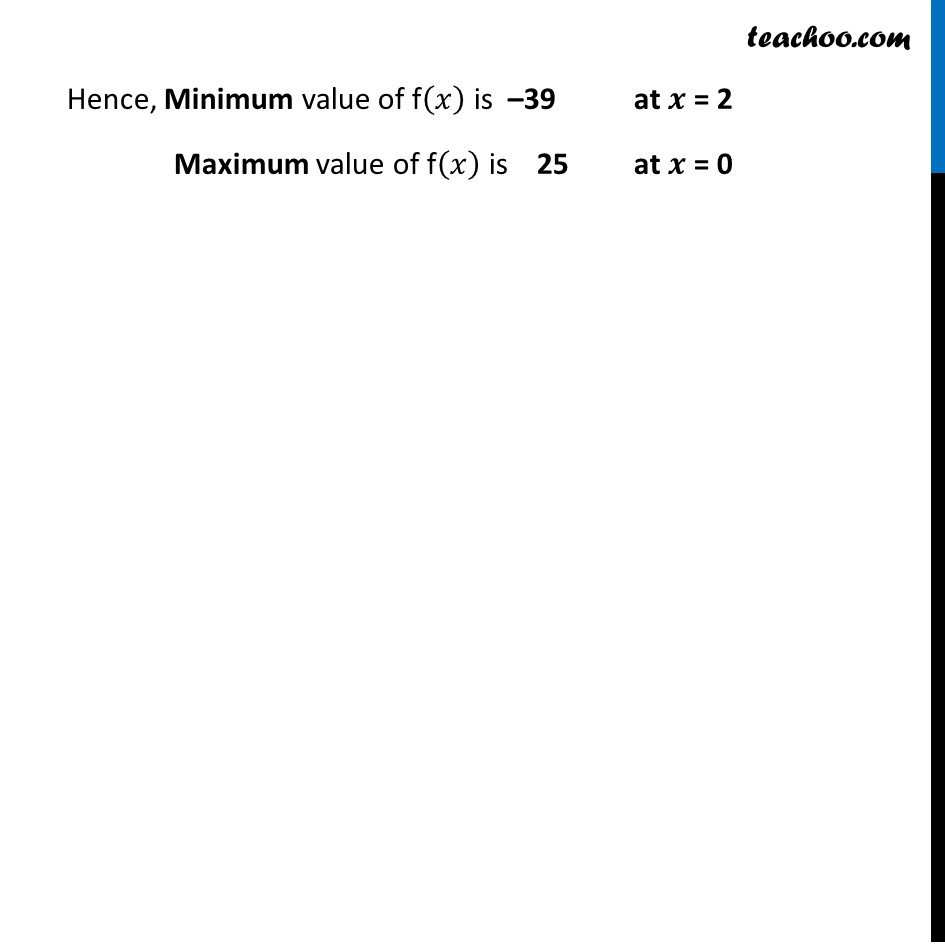Ex 6.3

Chapter 6 Class 12 Application of Derivatives
Serial order wiseLearn in your speed, with individual attention - Teachoo Maths 1-on-1 Class

### Transcript

Ex 6.3, 7 Find both the maximum value and the minimum value of 3𝑥4 – 8𝑥3 + 12𝑥2 – 48𝑥 + 25 on the interval [0, 3].Let f(x) = 3𝑥4 – 8𝑥3 + 12𝑥2 – 48𝑥 + 25, where 𝑥 ∈ [0, 3] Finding f’(𝒙) f’(𝑥)=𝑑(3𝑥^4 − 8𝑥^3 + 12𝑥^2 − 48𝑥 + 25)/𝑑𝑥 f’(𝑥)=3 ×4𝑥^3−8 ×3𝑥^2+12 ×2𝑥−48+0 f’(𝑥)=12𝑥^3−24𝑥^2+24𝑥−48 f’(𝑥)=12(𝑥^3−2𝑥^2+2𝑥−4) Putting f’(𝒙)=𝟎 12(𝑥^3−2𝑥^2+2𝑥−4)=0 𝑥^3−2𝑥^2+2𝑥−4=0 𝑥^2 (𝑥−2)+2(𝑥−2)=0 (𝑥^2+2)(𝑥−2)=0 Since 𝑥^2=−2 is not possible Thus 𝑥=2 is only critical point Since are given interval 𝑥 ∈ [0 , 3] Hence , calculating f(𝑥) at 𝑥 = 0 , 2 & 3 Hence, Minimum value of f(𝑥) is –39 at 𝒙 = 2 Maximum value of f(𝑥) is 25 at 𝒙 = 0